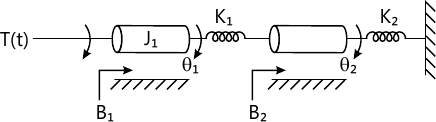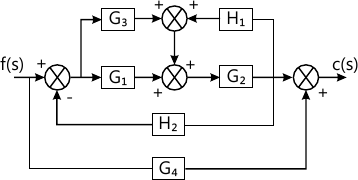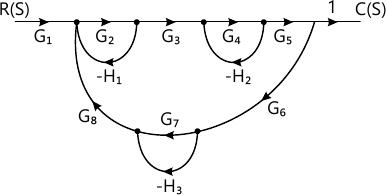Total marks: --
Total time: --
INSTRUCTIONS
(1) Assume appropriate data and state your reasons
(2) Marks are given to the right of every question
(3) Draw neat diagrams wherever necessary

1 (a) Explain with examples open loop and closed loop control system. List merits and demerits of both.
10 M
1 (b) Draw the electrical network based on torque-current analogy give all the performance equation for the Fig. Q1(b).10 M

2 (a) Obtain the T.F. of the system using block diagram reduction method.10 M
2 (b) Obtain the transfer function using signal flow graph. By Mason's gain formula.10 M

3 (a) Draw the transient response characteristics of a control system to a unit step input and define the following: i) Delay time; ii) Rise time; iii) Peak time; iv) Maximum overshoot; v) Settling time.
6 M
3 (b) Derive the expression for peak time tp for a second order system for step input.
4 M
3 (c) The response of a servo mechanism is c(t)=1+0.2e-50t-1.2e-10t when subjected to a unit step input. Obtain an expression for closed loop transfer function. Determine the un-damped natural frequency and damping ratio.
4 M
3 (d) The open loop transfer function of a unity feedback system is given by $G(s)= \dfrac {K}{S}(ST+1)$ where k and T are positive constant. By what factor should the amplifier, gain 'K' be reduced so that the peak, overshoot of unit step response of the system is reduced from 75% to 25%.
6 M

4 (a) Explain Routh-Hurwitz's criterion in stability of a control system.
4 M
4 (b) The characteristics equation for certain feedback control system are given below. Determine the system is stable or not and find the value of for a stable system S3+3ks2+(k+2)s+4=0
6 M
4 (c) The open loop T.F. of a unity feedback system is given by $G(s)= \dfrac {k(s+3)}{s(s^2+2s+3)(s+5)(s+6)}$ Find the value of 'K' of which the closed loop system is stable.
6 M
4 (d) What are the disadvantages of RH criterion on stability of control system?
4 M

5 (a) For a unity feedback system, the open-loop transfer function is given by $G(s)= \dfrac {K}{s(s+2)(s^2-6s+25)}$ i) Sketch the root locus for 0?K??
.ii) At what value of 'K' the system becomes unstable
iii) At this point instability, determine the frequency of oscillation of the system
15 M
5 (b) Consider the system with $G(s)H(s)= \dfrac {K}{s(s+2)(s+4)}$ find whether s- -.075 and s=-1+j4 is on the root locus or not using angle condition.
5 M

6 (a) Construct the Bode plots for a unity feedback control system having $G(s)= \dfrac {2000}{s(s+1)(s+100)}$ from the Bode plots determine;
i) Gain cross over frequency
ii) Phase cross over frequency
iii) Gain margin
iv) Phase margin
Comment on stability
14 M
6 (b) List the limitation of lead and lag compensations.
6 M

7 (a) The transfer function of a control system is given by $\dfrac {y(s)}{u(s)} = \dfrac {s^2+3s+4}{s^1+2s^2+3s+2}$ obtain a state model.
10 M
7 (b) State the properties of state transition matrix and derive them.
10 M

8 (a) Explain the procedure for investigation the stability using Nyquist criterion.
8 M
8 (b) Using Nyquist stability criterion, investigator the closed loop stability of a negative feedback control system whose open loop transfer function is given by $G(s)H(s)= \dfrac {K(ST_a+1)}{s^2}, K, T_2 >0.$
12 M

More question papers from Control Systems